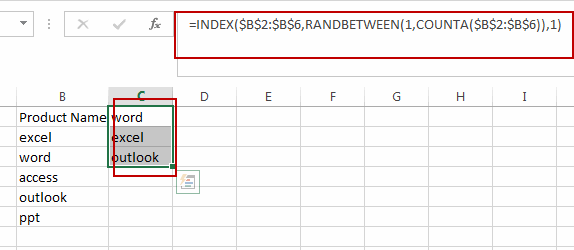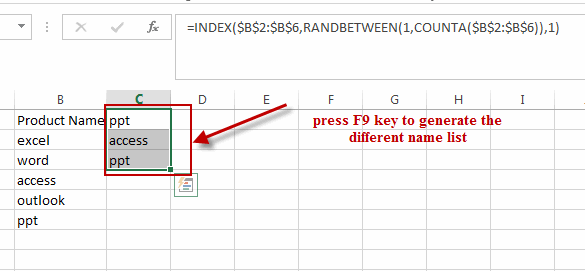# How to Pick a Random Name from a List in Excel

This post will guide you how to pick a random name from a list in Excel. How do I select random value from a list or a table with a formula in Excel 2013/2016.

## Pick Random Name from a List

Assuming that you have a list of names in range B1:B6, and you want to pick random names from this list. How to do it. You can use a formula based on the INDEX function, the RANDOMBETWEEN function and the COUNTA function to achieve the result. You can use one of the following formulas:

`=INDEX(\$B\$2:\$B\$6,RANDBETWEEN(1,COUNTA(\$B\$2:\$B\$6)),1)`

Or

`=INDEX(\$B\$2:\$B\$6,RANDBETWEEN(1,ROWS(\$B\$2:\$B\$6)),1)`

Type this formula into a blank cell and press Enter key on your keyboard. And then drag the Fill Handle down to list the random names that you need. And then press F9 key, it will generate the different name list randomly.Note: you should know that the name list will be changed when you refresh the current worksheet.

### Related Functions

• Excel INDEX function
The Excel INDEX function returns a value from a table based on the index (row number and column number)The INDEX function is a build-in function in Microsoft Excel and it is categorized as a Lookup and Reference Function.The syntax of the INDEX function is as below:= INDEX (array, row_num,[column_num])…
• Excel COUNTA function
The Excel COUNTA function counts the number of cells that are not empty in a range. The syntax of the COUNTA function is as below:= COUNTA(value1, [value2],…)…
• Excel RANDBETWEEN Function
The Excel RANDBETWEEN function returns a random integer number that is between the numbers you specify.The syntax of the RANDBETWEEN function is as below:= RANDBETWEEN (bottom,top)….

### You might also like:

Sidebar